# 06-240/Homework Assignment 9

Jump to: navigation, search

Read all of chapter 4 in our textbook. Remember that reading math isn't like reading a novel! If you read a novel and miss a few details most likely you'll still understand the novel. But if you miss a few details in a math text, often you'll miss everything that follows. So reading math takes reading and rereading and rerereading and a lot of thought about what you've read. Also, preread sections 5.1 and 5.2, just to get a feel for the future.

Solve problems 1, 2, 3, 6, 22 and 23 on pages 220-222 and problems 9, 11, 20, 21, 22 and 24 on pages 228-230 but submit only your solutions of the underlined problems. This assignment is due at the tutorials on Thursday November 30.

Just for fun. A certain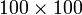$100\times 100$ matrix$A$ of random numbers between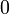$0$ and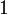$1$ is fed into a computer called Golem, capable of about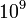$10^9$ arithmetic operations per second (between floating point numbers, at roughly 14 decimal digits of precision).

• Estimate how long it will take Golem to compute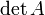$\det A$ using the explicit recursive formula.
• As you may know, glass is really a liquid and it slowly flows with gravity. How many times will you need to replace your computer screen before the computation is done?
• Assuming you are ready to wait and shuffle screens, will you trust the results? (Remember that even if electrical power will be available to eternity and electronic components will never fail, every time a computer adds or multiplies two 14-digit numbers it makes a rounding error of size around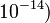$10^{-14})$.
• Estimate how long it will take Golem to compute$\det A$ using row operations.
• Assuming you are ready to wait, will you trust the results (remembering the same comment as above)? How many screens will you go through this time?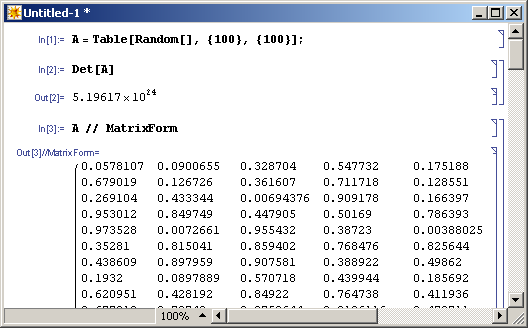Computed on Dror's laptop in a fraction of a second. The matrix is cropped, of course.